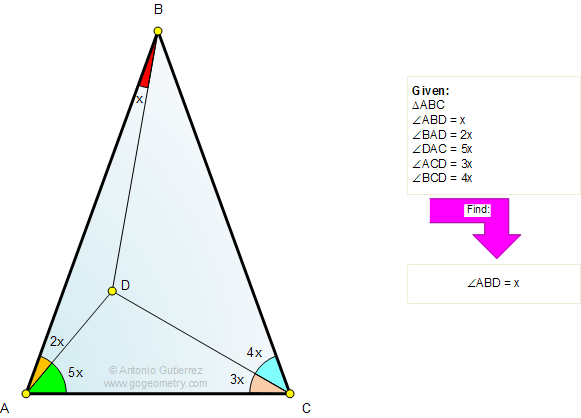# Online Geometry Problem 696: Triangle, Angles, Auxiliary Construction, Congruence. Level: High School, College, Mathematics Education

 The figure shows a triangle ABC with angle ABD = x, angle BAD = 2x, angle DAC = 5x, angle ACD = 3x, angle BCD = 4x. Find the measure of the angle ABD.Home | Search | Geometry | Problems | All Problems | Open Problems | Visual Index | Problems Art Gallery | 691-700 | How to Solve Problems | Triangles | Angles | Congruence | Email Solution / comment | By Antonio Gutierrez